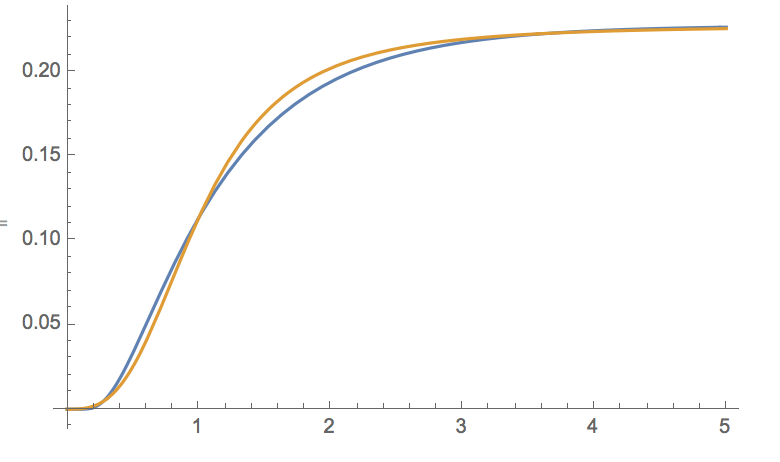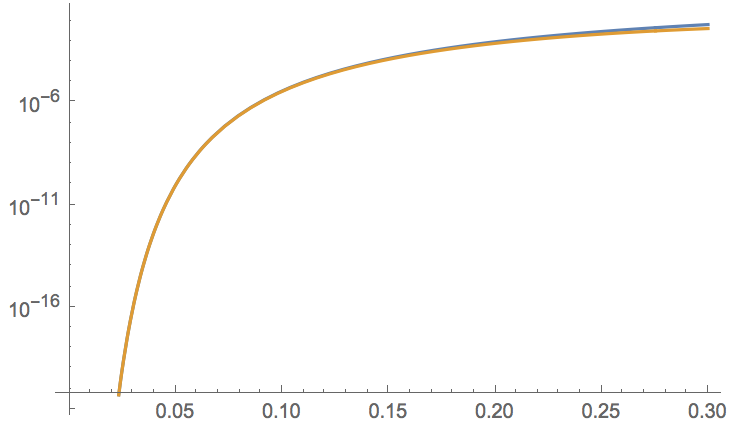# Is there a way to get the closed form approximate result of $\int_ 0^a\frac {e^{-x - \frac {1} {x}}} {x}\, dx$ [closed]

It is known that $$\int_ 0^{\infty}\frac {e^{-x - \frac {1} {x}}} {x} dx=2 K_0(2),$$ but now I want to get the closed form approximate result of $$\int_ 0^a\frac {e^{-x - \frac {1} {x}}} {x} dx.$$ I have searched the classic Table of Integrals, Series, and Products, but there is no pattern match this situation. Is there some approaches to the problem?

## closed as off-topic by Ryan Budney, Wolfgang, Stefan Kohl, Marco Golla, MyshkinDec 4 '16 at 19:54

This question appears to be off-topic. The users who voted to close gave these specific reasons:

• "MathOverflow is for mathematicians to ask each other questions about their research. See Math.StackExchange to ask general questions in mathematics." – Stefan Kohl, Myshkin
• "This question does not appear to be about research level mathematics within the scope defined in the help center." – Wolfgang, Marco Golla
If this question can be reworded to fit the rules in the help center, please edit the question.

• If you want large $a$, there is an asymptotic expansion for $\int_a^\infty$ that starts $e^{-a} (1/a-2/a^2+9/(2a^3)+\cdots)$. – Brendan McKay Dec 4 '16 at 3:43
• What range of $a$ did you have in mind? – Pat Devlin Dec 4 '16 at 5:47

## 1 AnswerThe blue curve is the desired integral $$\int_ 0^{a}\frac {e^{-x - \frac {1} {x}}} {x} dx$$, the orange curve is the approximate answer $$2K_0(2)a^3(1+a^3)^{-1}$$.

You could use this for a global approximation. In the small-$$a$$ region the asymptotics is $$e^{-1/a}(a-2a^2)$$, which is quite accurate up to about $$a=0.2$$, see plot below. Incidentally, the small-$$a$$ and large-$$a$$ asymptotics (see Brendan McKay's comment) are the same upon replacement of $$a$$ by $$1/a$$.